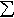#Interactive Real Analysis

Next | Previous | Glossary | Map

## 7.4. Lebesgue Integral

### Example 7.4.2(b): Simple Functions

Are simple functions uniquely determined? In other words, if s1 and s2 are two simple functions with s1(x) = s2(x), do they have to have the same representation? If different representations are possible, which one is "the best"?
No, two simple functions can be the same but have different representations. For example, take the sets
A = [0, 1] and B = (1, 2]
A' = AQ and A'' = AI
B' = BQ and B'' = BI
where Q are the rationals and I are the irrationals. Then all sets are measurable. Define two simple functions
s1(x) = XA(x) + XB(x)
s2(x) = XA' (x) + XA'' (x) + XB' (x) + XB'' (x)
Obviously the two simple functions have different representations, but s1(x) = s2(x) for all x. Note that s1 is a simple function as well as a step function, while s2 is not a step function.

Simple functions assume only finitely many values. If s is a simple function and { a1, ..., an } are the non-zero values of s, define the sets

Aj = { x: s(x) = aj }
Then the sets { Aj } are all disjoint and
s(x) =aj XAj(x)
Therefore, we can always assume without loss of generality that if s(x) =cj XEj(x) is any simple function, the sets Ej are disjoint and the numbers cj are not zero. This representation (the sets are disjoint and the coefficients are non-zero) is the canonical representation of a simple function.
Next | Previous | Glossary | Map